## Class 12-Maths

#### Class 12-Maths

Course Language : English

Total Duration : 150:30 Hours

Course Price : ₹ 6600

Maths can be broadly classified as:

• Algebra
• Applied Mathematics
• Calculus and Analysis
• Discrete Mathematics
• Foundations of Mathematics
• Geometry
• History and Terminology
• Number Theory
• Probability and Statistics
• Recreational Mathematics

#### Recruiters

Unit-I: Relations and Functions

1. Relations and Functions (15 Periods)

Types of relations: reflexive, symmetric, transitive and equivalence relations. One to one and onto functions, composite functions, inverse of a function.

2. Inverse Trigonometric Functions 15 Periods

Definition, range, domain, principal value branch. Graphs of inverse trigonometric

Functions Elementary properties of inverse trigonometric functions.

Unit-II: Algebra

1. Matrices (25 Periods)

Concept, notation, order, equality, types of matrices, zero and identity matrix, transpose of a matrix, symmetric and skew symmetric matrices. Operation on matrices: Addition and multiplication and multiplication with a scalar. Simple properties of addition, multiplication and scalar multiplication.

Non- commutativity of multiplication of matrices and existence of non-zero matrices whose product is the zero matrix (restrict to square matrices of order 2).Concept of elementary row and column operations. Invertible matrices and proof of the uniqueness of inverse, if it exists; (Here all matrices will have real entries).

2. Determinants (25 Periods)

Determinant of a square matrix (up to 3 x 3 matrices), properties of determinants, minors, co-factors and applications of determinants in finding the area of a triangle. Adjoint and inverse of a square matrix. Consistency, inconsistency and number of solutions of system of linear equations by examples, solving system of linear equations in two or three variables (having unique solution) using inverse of a matrix.

Unit-III: Calculus

1. Continuity and Differentiability (20 Periods)

Continuity and differentiability, derivative of composite functions, chain rule, derivative of inverse trigonometric functions, derivative of implicit functions. Concept of exponential and logarithmic functions.

Derivatives of logarithmic and exponential functions. Logarithmic differentiation, derivative of functions expressed in parametric forms. Second order derivatives. Rolle’s and Lagrange's Mean Value Theorems (without proof) and their geometric interpretation.

2. Applications of Derivatives (10 Periods)

Applications of derivatives: rate of change of bodies, increasing/decreasing functions, tangents and normals, use of derivatives in approximation, maxima and minima (first derivative test motivated geometrically and second derivative test given as a provable tool). Simple problems (that illustrate basic principles and understanding of the subject as well as real-life situations).

3. Integrals (20 Periods)

Integration as inverse process of differentiation.Integration of a variety of functions by substitution, by partial fractions and by parts, Evaluation of simple integrals of the following types and problems based on them.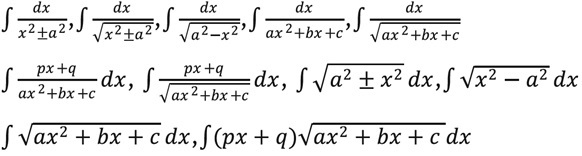Definite integrals as a limit of a sum, Fundamental Theorem of Calculus (without proof).Basic properties of definite integrals and evaluation of definite integrals.

4. Applications of the Integrals (15 Periods)

Applications in finding the area under simple curves, especially lines, circles/ parabolas/ellipses (in standard form only), Area between any of the two above said curves (the region should be clearly identifiable).

5. Differential Equations (15 Periods)

Definition, order and degree, general and particular solutions of a differential equation. formation of differential equation whose general solution is given. Solution of differential equations by method of separation of variables, solutions of homogeneous differential equations of first order and first degree. Solutions of linear differential equation of the type:

(dy/dx) + py = q, where p and q are functions of x or constants.

(dx/dy) + px = q, where p and q are functions of y or constants.

Unit-IV: Vectors and Three-Dimensional Geometry

1. Vectors (15 Periods)

Vectors and scalars, magnitude and direction of a vector. Direction cosines and direction ratios of a vector. Types of vectors (equal, unit, zero, parallel and collinear vectors), position vector of a point, negative of a vector, components of a vector, addition of vectors, multiplication of a vector by a scalar, position vector of a point dividing a line segment in a given ratio. Definition, Geometrical

Interpretation, properties and application of scalar (dot) product of vectors, vector (cross) product of vectors, scalar triple product of vectors.

2. Three - dimensional Geometry (15 Periods)

Direction cosines and direction ratios of a line joining two points. Cartesian equation and vector equation of a line, coplanar and skew lines, shortest distance between two lines. Cartesian and vector equation of a plane.Angle between (i) two lines, (ii) two planes, (iii) a line and a plane. Distance of a point from a plane.

Unit-V: Linear Programming

1. Linear Programming (20 Periods)

Introduction, related terminology such as constraints, objective function, optimization, different types of linear programming (L.P.) problems, mathematical formulation of L.P. problems, graphical method of solution for problems in two variables, feasible and infeasible regions (bounded or unbounded), feasible and infeasible solutions, optimal feasible solutions (up to three non-trivial constraints).

Unit-VI: Probability

1. Probability (30 Periods)

Conditional probability, multiplication theorem on probability, independent events, total probability, Bayes’ theorem, Random variable and its probability distribution, mean and variance of random variable.

The study of angles and of the angular relationships of planar and three-dimensional figures is known as trigonometry. The trigonometric functions (also called the circular functions) comprising trigonometry are the cosecant cscx, cosine cosx, cotangent cotx, secant secx, sine sinx, and tangent tanx.

The trigonometric functions are most simply defined using the unit circle. Letbe an angle measured counterclockwise from the x-axis along an arc of the circle. Then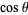is the horizontal coordinate of the arc endpoint, and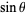is the vertical component. The ratio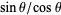is defined as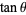. As a result of this definition, the trigonometric functions are periodic with period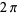, so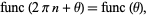(1)

whereis an integer and func is a trigonometric function.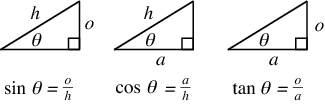###### Student Reviews
•###### Godwin Joe
October 11, 2019

He’s absolutely awesome at teaching but sometimes the course matter seems a little bit rushed. This is a rare occurrence but I did find myself hung up a couple of times due to this.

/ 5 Star
•###### Tania Tania
October 03, 2019

He’s absolutely awesome at teaching but sometimes the course matter seems a little bit rushed. This is a rare occurrence but I did find myself hung up a couple of times due to this.

/ 5 Star

#### Related CoursesCourse
NEET+KCETCourse